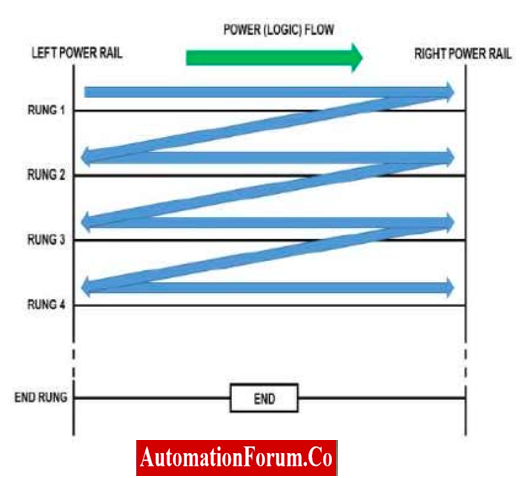# Equivalent Logic gates used in PLC Ladder Diagram• It is most popular programming language for PLC.
• It resemble the hard wired relay logic
• Ladder diagram has two vertical lines representing two power rails to which the circuits are connected.
• The horizontal line is called rung and each rung represent the control process operation
• A ladder diagram is read from left to right and top to bottom as shown.
• If power flow is on then the output coil is in on state

## Basic Symbols in Ladder Diagram

The PLC logic ladder diagram is represented as shown below. (Rung with NO, NC contacts and output coil)

## What logic gates are used in ladder diagram?

Below mentioned logics are normally used in PLC Ladder logic

• AND gate
• OR gate
• NOT gate
• NAND gate
• NOR gate
• EX-OR gate
• EX-NOR gate

### Explanation of Program

IF Sensor_1 AND Sensor_2 THEN

SOL_1:=1;

ELSEIF Sensor_3 AND Sensor_4 AND NOT Sensor_5 THEN

SOL_1=1;

END_IF;

## What is AND LOGIC GATE & PLC LADDER DIAGRAM?

• If all inputs is high the output is high
• If any of the inputs are low then output is low

## What is OR LOGIC GATE & PLC LADDER DIAGRAM?

• If any input is high the output is high
• If all the inputs are low then output is low

## What is NOT LOGIC GATE & PLC LADDER DIAGRAM?

• NOT gate denotes inverted process
• If input is high the output is low
• If input is low then output is high

## What is NAND LOGIC GATE & PLC LADDER DIAGRAM?

• NAND gate is inverted AND gate.
• If any input is high the output is high
• If all inputs are low then output is high

## What is NOR LOGIC GATE & PLC LADDER DIAGRAM?

• NOR gate is inverted OR gate.
• If all inputs are low the output is high
• If any input is high then output is low

## What is EX-OR LOGIC GATE & PLC LADDER DIAGRAM?

• If all inputs are different the output is high
• If al inputs are same then output is low

## What is EX-NOR LOGIC GATE & PLC LADDER DIAGRAM?

• If all inputs are different the output islow
• If all inputs are same then output is high